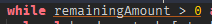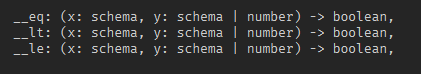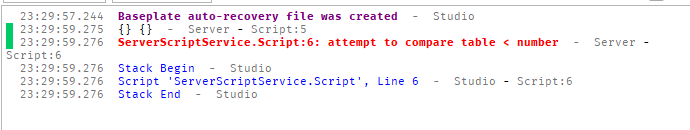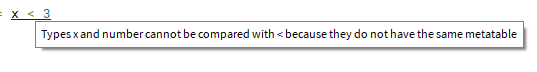# Metamethod __lt, __eq, __le show an error when not provided identical types

Metamethod __lt, __eq, __le show an error when not provided identical types even though the type implementation in the metatable allows for differing types.Should allow for differing types. Our use case is a Big Integer class.

2 Likes

Luau doesn’t support this. In order to compare two objects they need to have the same metatable method.

``````local x = setmetatable({}, {
__lt = print,
})

local _ = x < x -- Will print something
local _ = x < 3 -- Error: attempt to compare table < number
``````
1 Like

Clearly. I am asking for support for it. The VM itself DOES support this behavior, however it is being incorrectly shown as an error in studio.

That error is from the runtime. Check again.The error Luau gives you also explains this:The wiki as well:

You are correct, this is an error in runtime. Unfortunate.

``````--!strict

export type metatable_schema = {
__eq: (self: schema, operand: schema | Vector2) -> boolean,
__lt: (self: schema, operand: schema | Vector2) -> boolean,
__le: (self: schema, operand: schema | Vector2) -> boolean,
}

export type raw_schema = {
X: number,
Y: number,
}

export type schema = typeof(setmetatable({}::raw_schema, {}::metatable_schema))

local metatable = {}

function metatable.__eq(self: schema, operand: schema | Vector2): boolean
return self.X == operand.X and self.Y == operand.Y
end

function metatable.__lt(self: schema, operand: schema | Vector2): boolean
return self.X * self.Y < operand.X * operand.Y
end

function metatable.__le(self: schema, operand: schema | Vector2): boolean
return self.X * self.Y <= operand.X * operand.Y
end

function MyVector2(
x: number,
y: number
): schema
return setmetatable({ X = x, Y = y }, metatable)
end

local a = Vector2.new(1, 2)
local b = MyVector2(1, 2)

pcall(function()
print(b < a)
print("b < a")
end)
pcall(function()
print(b > a)
print("b > a")
end)
pcall(function()
print(b <= a)
print("b <= a")
end)
pcall(function()
print(b >= a)
print("b >= a")
end)

pcall(function()
print(a < b)
print("a < b")
end)
pcall(function()
print(a > b)
print("a > b")
end)
pcall(function()
print(a <= b)
print("a <= b")
end)
pcall(function()
print(a >= b)
print("a >= b")
end)

pcall(function()
print(a == b)
print("a == b")
end)
pcall(function()
print(b == a)
print("b == a")
end)

return module
``````